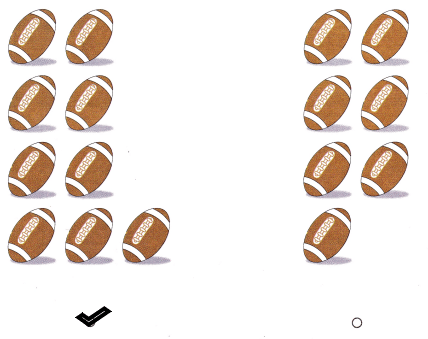# Texas Go Math Kindergarten Lesson 5.2 Answer Key Count and Write 9

Refer to our Texas Go Math Kindergarten Answer Key Pdf to score good marks in the exams. Test yourself by practicing the problems from Texas Go Math Kindergarten Lesson 5.2 Answer Key Count and Write 9.

## Texas Go Math Kindergarten Lesson 5.2 Answer Key Count and Write 9

Explore

DIRECTIONS: Count and tell how many cubes. Write the numbers. Count and tell how many ducks. Trace the word.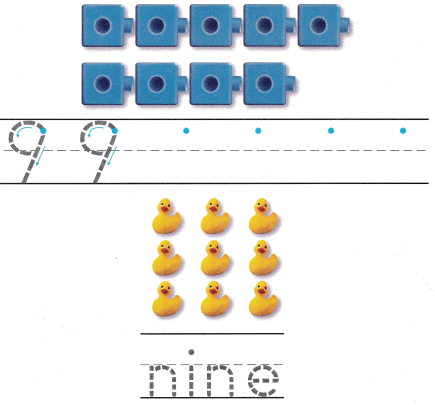Explanation:
I traced and practiced writing the number 9 and traced the word nine.

Share and Show

DIRECTIONS: 1. Look at the picture. Circle the sets of nine objects.

Question 1.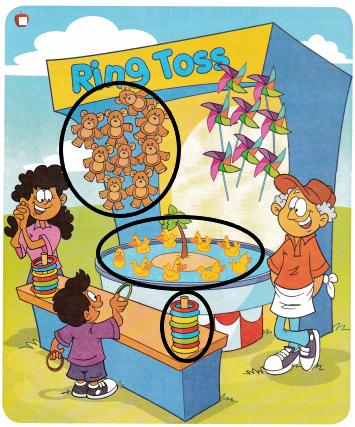Explanation:
I drew a circle around those groups that are 9 in number.There are 9 teddy bears, 9 ducks and 9 rings.

DIRECTIONS: 2. Say the number. Write the numbers. 3-6. Count and tell how many. Write the number.

Question 2.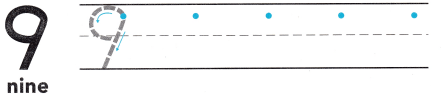Explanation:
I traced and practiced writing the number 9.

Question 3.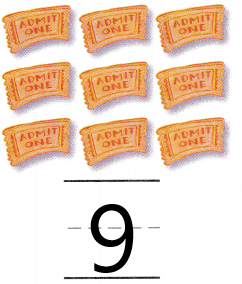Explanation:
I counted and wrote the number 9 as there are 9 objects.

Question 4.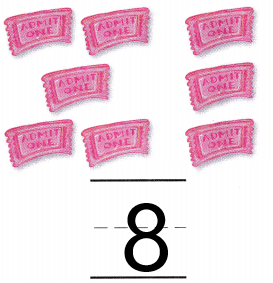Explanation:
I counted and wrote the number 8 as there are 8 objects.

Question 5.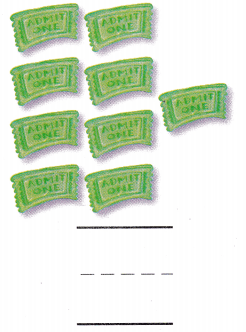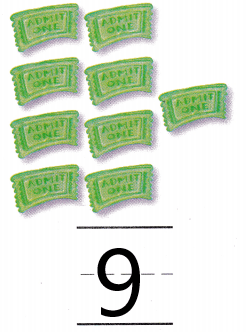Explanation:
I counted and wrote the number 9 as there are 9 objects.

Question 6.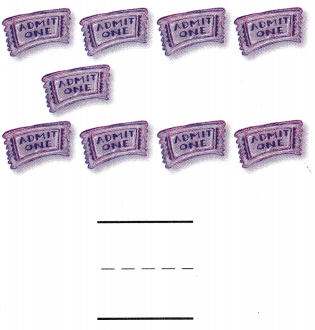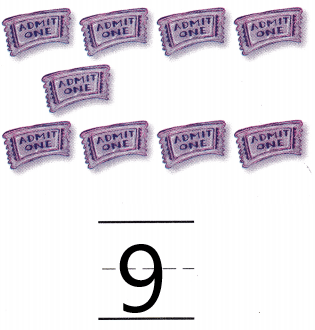Explanation:
I counted and wrote the number 9 as there are 9 objects.

HOME ACTIVITY • Ask your child to find something in your home that has the number 9 on it, such as a clock or a phone.

DIRECTIONS: 7. Which set has a number of objects one less than 10? Circle that set. 8. Choose the correct answer. Which set has nine ducks?

Problem Solving

Question 7.Explanation:
I drew a circle around the set that has one lessthan 10 that is 9.there are 9 brown bears.

Question 8.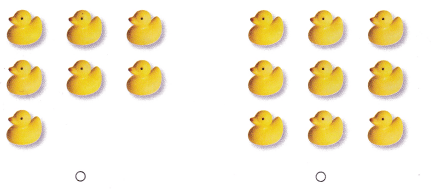Explanation:
I marked that group of ducks that are 9 in number.

### Texas Go Math Kindergarten Lesson 5.2 Homework and Practice Answer Key

DIRECTIONS: 1-4. Count and tell how many. Write the number.

Question 1.Explanation:
I counted and wrote the number 8 as there are 8 umbrellas.

Question 2.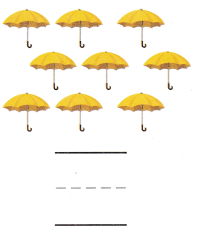Explanation:
I counted and wrote the number 9 as there are 9 umbrellas.

Question 3.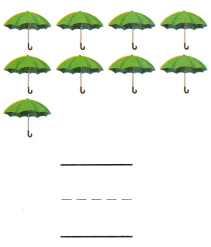Explanation:
I counted and wrote the number 9 as there are 9 umbrellas.

Question 4.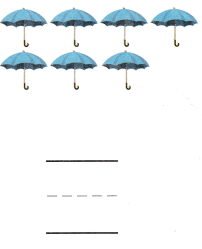Explanation:
I counted and wrote the number 7 as there are 7 umbrellas.

DIRECTIONS: Choose the correct answer. 5. Which set has 9 hats? 6. Which set has 9 footballs?

Lesson Check

Question 5.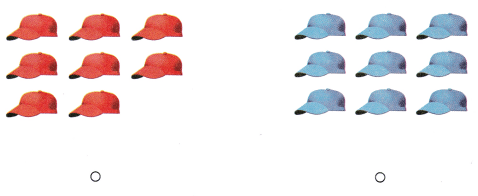Explanation:
I marked the set of hats that are 9 in number.

Question 6.